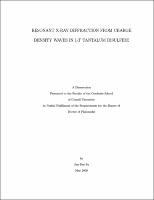## RESONANT X-RAY DIFFRACTION FROM CHARGE DENSITY WAVES IN 1-T TANTALUM DISULFIDEBy tuning the incident x-ray energy close to the Ta-L$_3$ edge, we studied resonant (elastic) x-ray diffraction (RXD) from the charge density waves (CDWs) of 1$T$-TaS$_2$. Our goal was to separate the scattering from the periodic modulation of the conduction electron density and from that of the lattice distortion wave. In addition to resonant diffraction studies, various x-ray techniques, including XANES, polarization analysis, and a temperature study, were utilized. We find that two physical effects prevent separating the CDW charge modulation scattering at the primary CDW satellites $\mathbf{G}\mp\mathbf{k}_{\mbox{\tiny{CDW}}}$ using energy or polarization. (i) The core-hole lifetime of the Ta-L$_3$ resonance is much larger than the CDW band gap in 1$T$-TaS$_2$ and smears out the CDW anomaly in the electronic density of states. (ii) Resonant scattering from Ta 5$d$ band states not associated with the CDW dominates over resonant scattering from the charge modulation, smearing out the polarization signature. Our results highlight the principles of RXD when the technique is used to study novel states found in the conduction bands of transition metal compounds and point out which types of systems are most promising.
Resonant x-ray diffraction; Charge density wave; XANES; Ta-L$_3$ edge; 1$T$-TaS$_2$